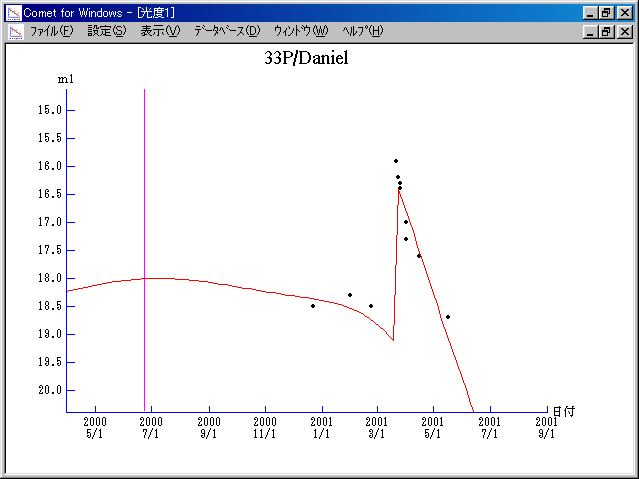# \$B%@%K%(%kWB@1(B

33P/Daniel (2000)###\$B50F;MWAG(B

```   The following improved orbital elements by Kenji Muraoka,
are from 60 observations 1979 to 2001, including 9 Planets,
Moon and 5 minor planets perturbations and non-gravitational
effect of style II. The mean residual is +/- 0.88 arc seconds.

Epoch  =  2000 June 25.0  TT       JDT = 2451720.5
T  =  2000 June 23.46617       +/- 0.01221 (m.e.) TT
Peri. =   18.98768                +/- 0.00074
Node  =   66.58265                +/- 0.00017   (2000.0)
Incl. =   22.41004                +/- 0.00011
q  =    2.1572385              +/- 0.0000139 AU
e  =    0.4635952              +/- 0.0000031
a  =    4.0216611              +/- 0.0000087 AU
n  =    0.12220694             +/- 0.00000040
P  =    8.065                  +/- 0.0000262  years
A1  =   +0.295                  +/- 0.025
A2  =   +0.07272                +/- 0.00093
```

###\$B@1?^(B###\$B8wEYJQ2=(B

```        m1 =  10.5 + 5 log\$B&\$(B + 15.0 log r  [   ,270]  (              \$B!A(B2001\$BG/(B03\$B7n(B20\$BF|(B)
m1 = -13.5 + 5 log\$B&\$(B + 60   log r  [270,380]  (2001\$BG/(B03\$B7n(B20\$BF|!A(B2001\$BG/(B07\$B7n(B08\$BF|(B)
m1 =  10.5 + 5 log\$B&\$(B + 15.0 log r  [380,   ]  (2001\$BG/(B07\$B7n(B08\$BF|!A(B              )
```##### \$B50F;MWAG\$OB<2,7r<#;a\$N7W;;\$K\$h\$k\$b\$N\$G\$9!#(B \$B@1?^\$O(B StellaNavigator Ver.2.0 for Windows (\$B%"%9%H%m%"!<%D(B \$BJTCx(B / \$B%"%9%-!<=PHG6I4)(B) \$B\$G:n@.\$7\$?\$b\$N\$G\$9!#(B \$B8wEY%0%i%U\$O(BComet for Windows\$B\$G:n@.\$7\$?\$b\$N\$G\$9!#(B